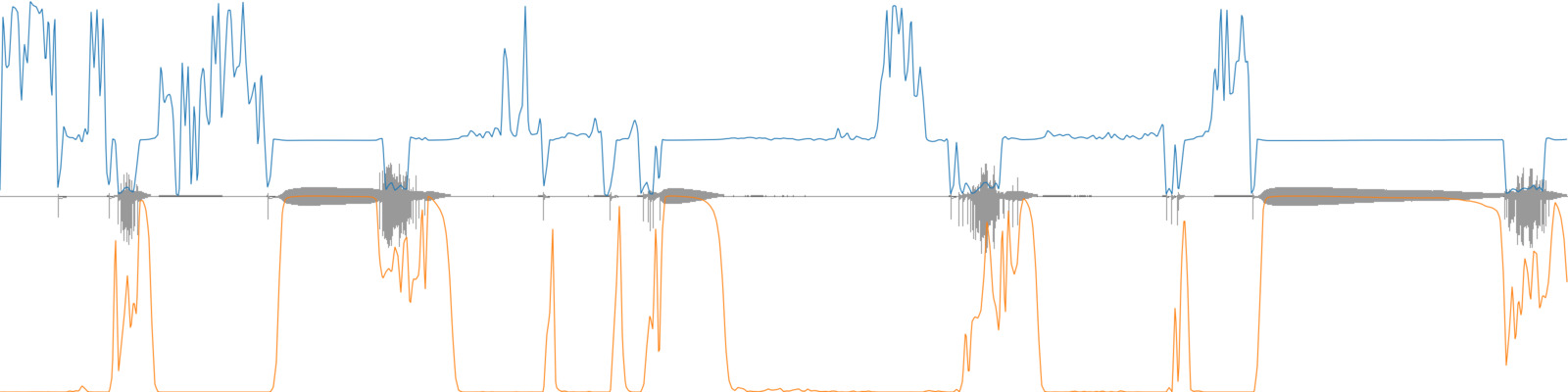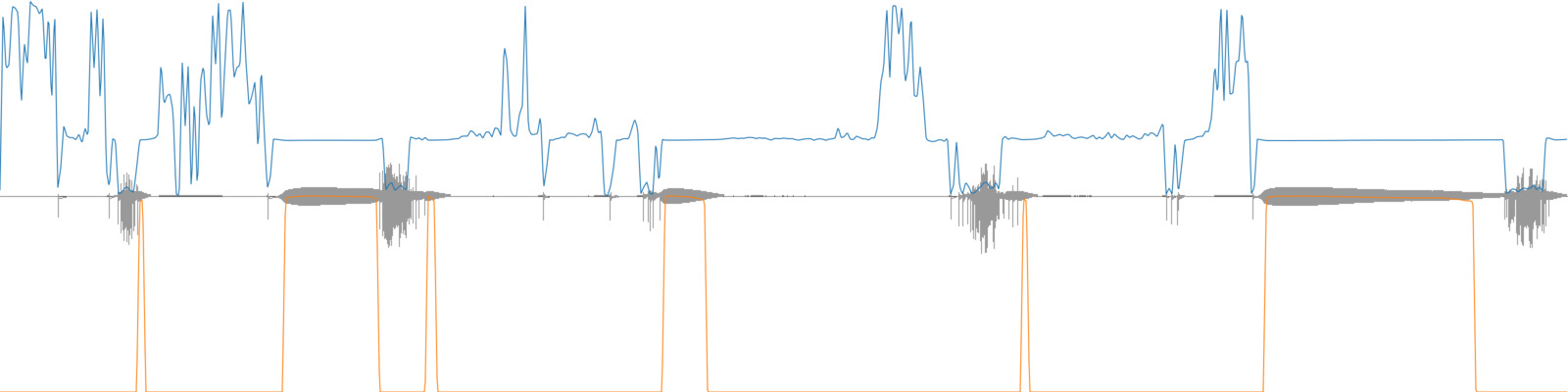# Weighting Stats

Apply weights to BufStats

The `weights` parameter of BufStats can be passed a buffer, the values of which will be used for relative weighting of each corresponding frame in the `source` buffer. This will create “weighted” statistics where some values in the data have more impact on the resulting statistical summary than others. All seven of the resulting statistics of BufStats may be affected by using the weights.

This can be useful for weighting certain moments in descriptor time-series by the value of other descriptors. For example, one might want to weight a descriptor time-series (such as MFCC) by amplitude so the louder moments of the sound slice have greater impact than the quieter moments on the resulting statistical summary.

Not providing a `weights` buffer will cause all the frames to be considered equally. Any negative values in the `weights` buffer will be treated as a weight of 0. The provided `weights` buffer must be a single channel with exactly the same number of frames as `source` (each frame in `weights` will be the weight amount for the corresponding frame in `source`).

## A musical example

Consider trying to determine the frequency of the tone in this recording.

A source recording with some clear tone, some silence, and some scratchy timbres.

The pitch analysis of this sound file shows a quite erratic time series, but with some moments of stable pitch.

As a first attempt, one might use BufStats to find the mean frequency of this pitch analysis, which is about 3,196 Hz. When a sine tone at this frequency is played alongside the source, we can hear that it isn’t very accurate.

Source recording with a sine tone at the mean frequency, as determined by BufStats.

### Finding a weighted mean of pitch using pitch confidence

The pitch analysis also provides a measure of pitch confidence, which ranges from 0 (less confident) to 1 (more confident), indicating how confident the algorithm is in the pitch it is returning. Looking at both, one can see that there are moments when the pitch confidence (orange) is quite high and the pitch analysis (blue) is quite steady.Source recording with the pitch analysis (blue) and pitch confidence (orange) overlaid.

By using the pitch confidence as the weights for BufStats the mean pitch value it returns will be a weighted mean, meaning that the frequency estimates when the algorithm is more confident will have a greater impact on the output mean pitch.

This weighted mean returned by BufStats is about 2,533 Hz. When a sine tone at this frequency is played alongside the source, we can hear that it isn’t much better, now it is too low!

Source recording with a sine tone at the weighted mean frequency, as determined by BufStats using pitch confidence for the weights.

### Using BufThresh to refine the weighting

Using BufThresh we can set any pitch confidence values below a threshold to zero. The weights of zero will ensure that the pitch analyses at those moments will not contribute to the calculation of the weighted mean pitch. Now only the moments where pitch confidence is above the threshold will be used to calculate the weighted mean pitch.Source recording with the pitch analysis (blue) and pitch confidence (orange) overlaid, where any pitch confidence values below 0.97 have been set to zero.

By thresholding the pitch confidence values at 0.97 and using these values for the weights, the weighted mean is now about 2,783 Hz. When a sine tone at this frequency is played alongside the source, we can hear that it is now quite close!

Source recording with a sine tone at the weighted mean frequency, as determined by BufStats using pitch confidence for the weights, where any pitch confidence values below 0.97 are set to zero.